# General Topology EntityStore

Entity store representing definitions and theorems of point-set topology

Originator: James Munkres

## Examples

### Basic Examples

Retrieve the ResourceObject:

 In:=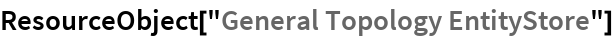Out=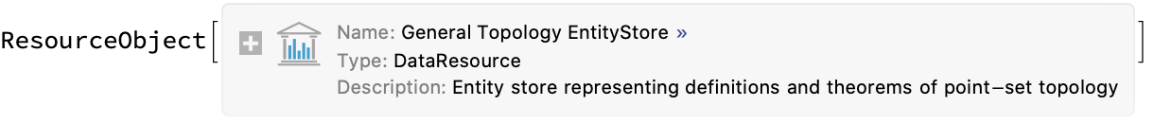View the data:

 In:=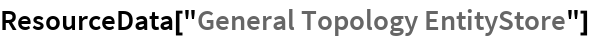Out=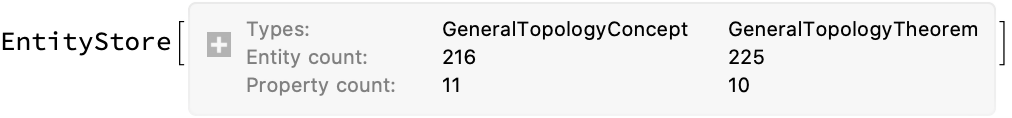Register the EntityStore:

 In:=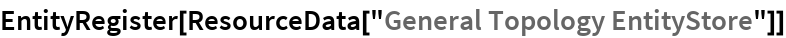Out=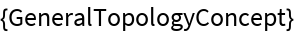In:=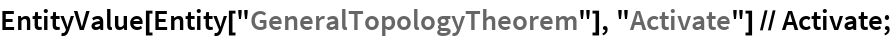In:=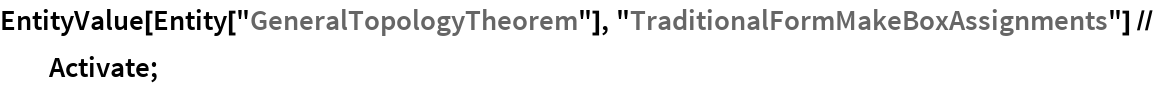In:=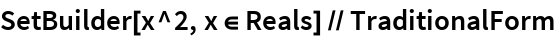Out=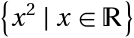View random concepts:

 In:=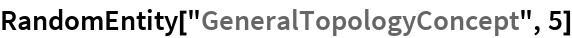Out=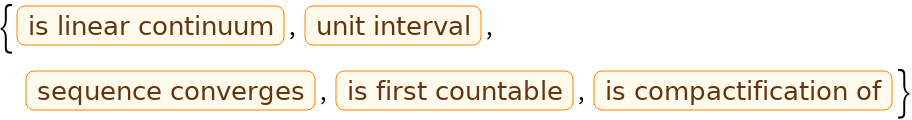View random theorems:

 In:=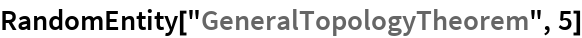Out=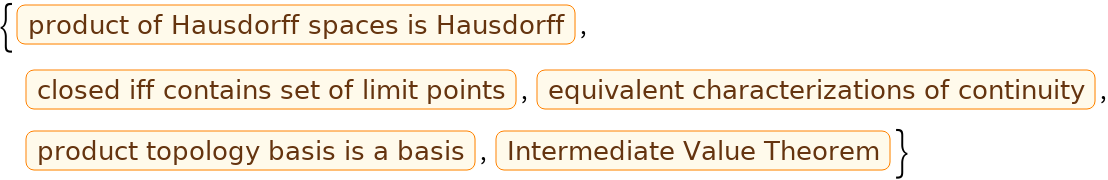### Visualization

View full theorem statements:

 In:=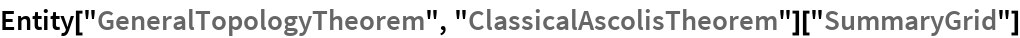Out=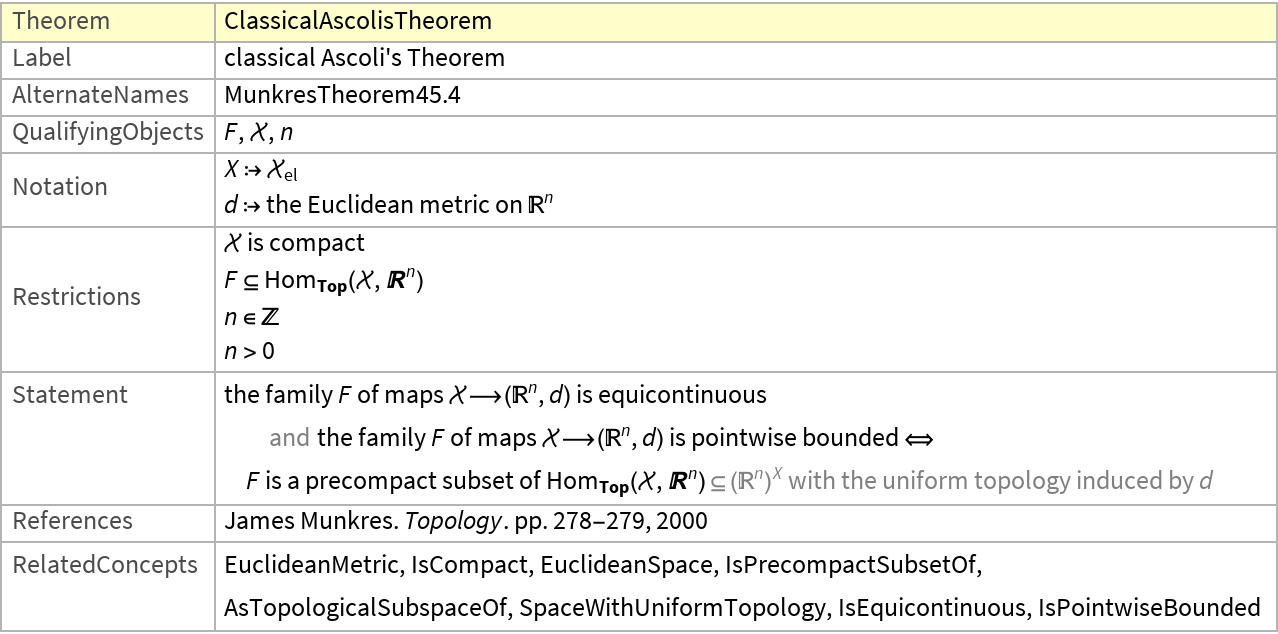View relationships between concepts and theorems:

 In:=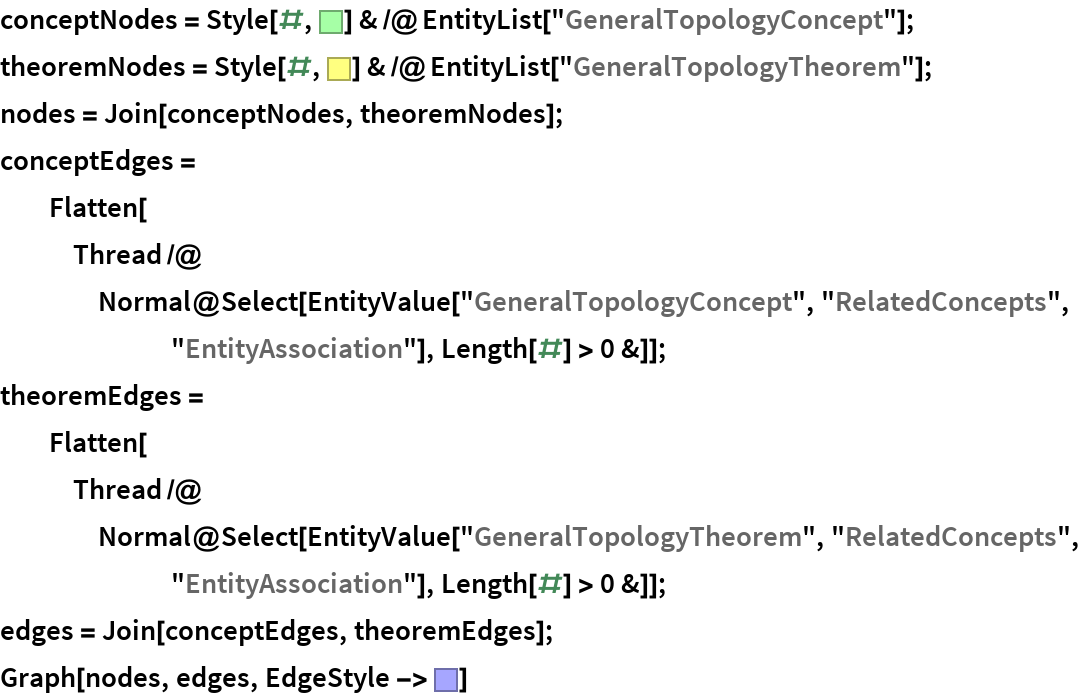Out=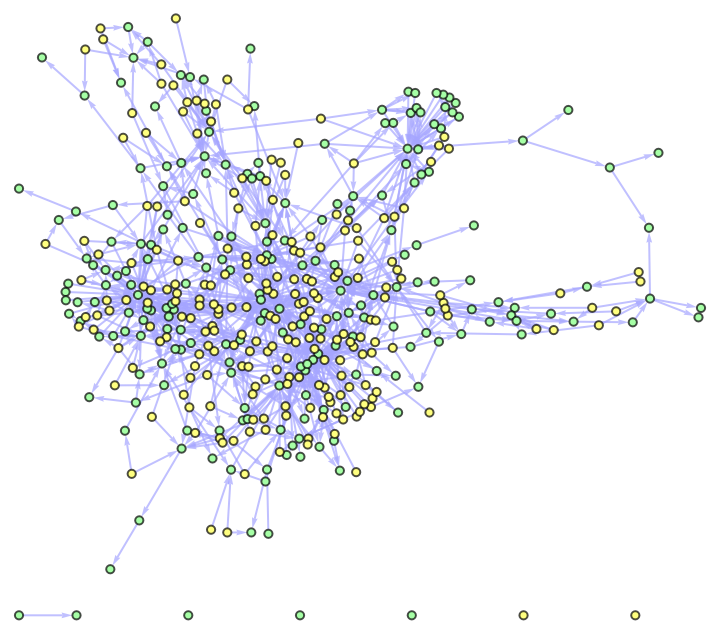Wolfram Research, "General Topology EntityStore" from the Wolfram Data Repository (2016) https://doi.org/10.24097/wolfram.56398.data